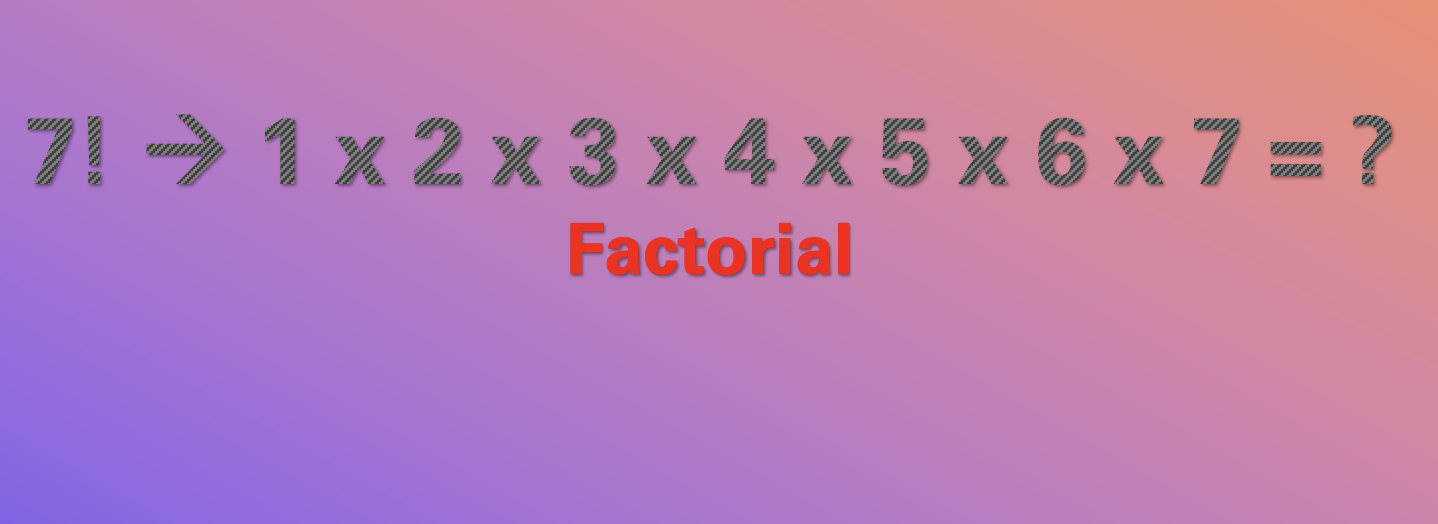# 17: Find Factorial of a Number - 1000+ Python Programs## What is a Factorial?

A Factorial is a mathematical operation used to calculate the product of all positive integers up to a given number.

For example, the factorial of 5 (written as 5!) is 1 x 2 x 3 x 4 x 5, which equals 120.

7! = 1 x 2 x 3 x 4 x 5 x 6 x 7 = 5040

## Pseudo Code

``````1. First, we get a number as input from the user.
2. Next, we initialize a variable factorial and set its value as 1.
3. We make use of the for loop to iterate from 1 to the input number.
4. While looping we multiply each number by the current value of factorial and store it back in factorial.
5. Finally, we print the value of the factorial variable which is our result.``````

## Python Code

``````# Python Program no. 17
# Find the Factorial of a Number
# 1000+ Python Programs by Code2care.org

# Get a number from the user
user_input_number = int(input("Enter a number: "))

# Initialize the factorial variable to 1
factorial = 1

# Calculate the factorial using a for loop
for i in range(1, user_input_number + 1):
factorial = factorial * i

# Print the result
print(f"The factorial of {user_input_number} is {factorial}")``````
Run the Program:

Enter a number: 7
The factorial of 7 is 5040

## Python Doc links for functions/concepts used:

-

Have Questions? Post them here!